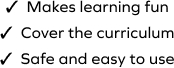# Understand Decimal Point Using Multiplication

SplashLearn - The complete PreK - Grade 5 Math & ELA Learning Program Built for Your Child
Home > Understand Decimal Point Using Multiplication
Kids often develop misconceptions about concepts in mathematics, including decimal. It is important to help them get over those misconceptions. The game involves working with a set of problems on decimal multiplication, where students relate multiplication of two decimals with the multiplication of two whole numbers and then place the decimal point at the correct place in the product. This game will nudge your fifth grader towards mastery and develop their all-round mathematical acumen.